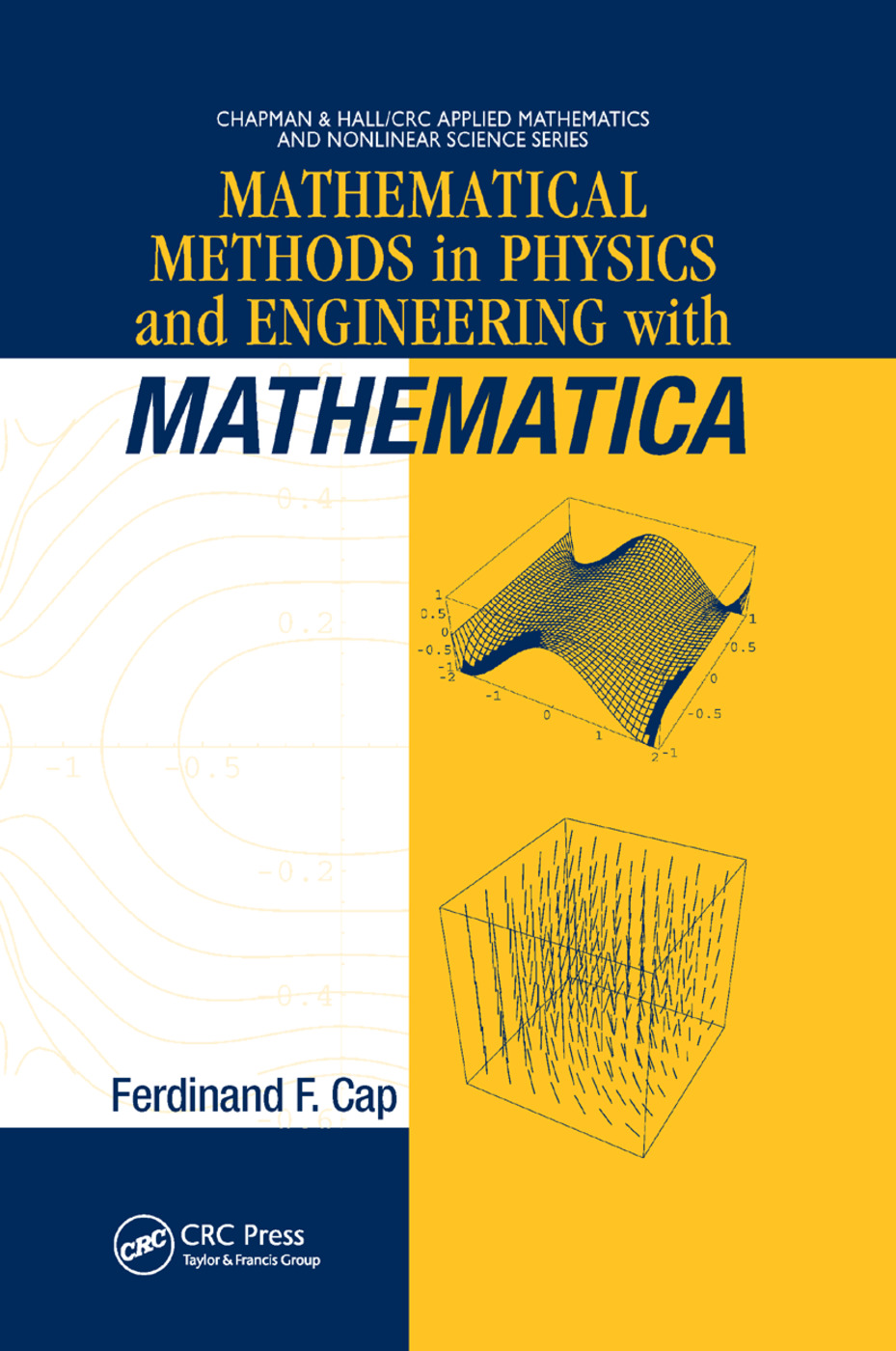Mathematical Methods in Physics and Engineering with Mathematica

1st Edition

CRC Press

352 pages | 50 B/W Illus.

Purchasing Options:\$ = USD
Paperback: 9780367395186
pub: 2019-09-11
SAVE ~\$14.99
\$74.95
\$59.96
x
Hardback: 9781584884026
pub: 2003-05-28
SAVE ~\$37.00
\$185.00
\$148.00
x
eBook (VitalSource) : 9780429210631
pub: 2003-05-28
from \$28.98

FREE Standard Shipping!

Description

More than ever before, complicated mathematical procedures are integral to the success and advancement of technology, engineering, and even industrial production. Knowledge of and experience with these procedures is therefore vital to present and future scientists, engineers and technologists.

Mathematical Methods in Physics and Engineering with Mathematica clearly demonstrates how to solve difficult practical problems involving ordinary and partial differential equations and boundary value problems using the software package Mathematica (4.x). Avoiding mathematical theorems and numerical methods-and requiring no prior experience with the software-the author helps readers learn by doing with step-by-step recipes useful in both new and classical applications.

Mathematica and FORTRAN codes used in the book's examples and exercises are available for download from the Internet. The author's clear explanation of each Mathematica command along with a wealth of examples and exercises make Mathematical Methods in Physics and Engineering with Mathematica an outstanding choice both as a reference for practical problem solving and as a quick-start guide to using a leading mathematics software package.

Reviews

"It is a whirlwind review of mathematical methods used to solve linear and nonlinear boundary-value problems. The best aspect of this book is its immersion in Mathematica, which is treated as a full partner in presenting the material. …A list of codes…and an appendix that lists all of the Mathematica commands used in the text are nice touches. …It will appeal to those who are looking for new perspectives, especially with Mathematica, on familiar topics that they learned elsewhere. It could also be used as a supplementary text in a course which uses an older, standard textbook."

-SIAM Review, Dean Duffy, Annapolis, Maryland

"This book is a good example of how to explain and how to use the Mathematica functions and add-on packages for solving various physical and engineering problems from the simplest to the most difficult ones. It is a new type of book, which provides an accessible guide for doing computer aided mathematics."

-Zentralblatt MATH 1044

INTRODUCTION

What is a Boundary Problem?

Classification of Partial Differential Equations

Types of Boundary Conditions and the Collocation Method

Differential Equations as Models for Nature

BOUNDARY PROBLEMS OF ORDINARY DIFFERENTIAL EQUATIONS

Linear Differential Equations

Solving Linear Differential Equations

Differential Equations of Physics and Engineering

Boundary Value Problems and Eigenvalues

Boundary Value Problems as Initial Value Problems

Nonlinear Ordinary Differential Equations

Solutions of Nonlinear Differential Equations

PARTIAL DIFFERENTIAL EQUATIONS

Coordinate Systems and Separability

Methods to Reduce Partial to Ordinary Differential Equations

The Method of Characteristics

Nonlinear Partial Differential Equations

BOUNDARY PROBLEMS WITH ONE CLOSED BOUNDARY

Laplace and Poisson Equations

Conformal Mapping in Two and Three Dimensions

d'Alembert Wave Equation and String Vibrations

Helmholtz Equation and Membrane Vibrations

Rods and the Plate Equation

Approximation Methods

Variational Calculus

Collocation Methods

BOUNDARY PROBLEMS WITH TWO CLOSED BOUNDARIES

Inseparable Problems

Holes in the Domain. Two Boundaries Belonging to Different Coordinate Systems

Corners in the Boundary

NONLINEAR BOUNDARY PROBLEMS

Some Definitions and Examples

Moving and Free Boundaries

Waves of Large Amplitudes. Solitons

The Rupture of an Embankment-Type Water Dam

Gas Flow with Combustion

REFERENCES

APPENDIX

INDEX

Cap, Ferdinand F.